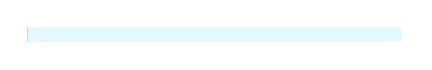# Appearance in Linear ProgressBar

7 Dec 20208 minutes to read

You can highly customize the appearance of the linear progressbar in the following ways.

The IndicatorCornerRadius property is used to frame rounded edges in the linear progressbar as demonstrated in the following code sample.

``<Syncfusion:SfLinearProgressBar Progress="70" IndicatorCornerRadius="10"/>``
``````SfLinearProgressBar linear = new SfLinearProgressBar { Progress = 70, IndicatorCornerRadius=10};
linear.Width = 500;
linear.Height = 20;Padding helps to generate space between track bar and progressbar in the linear progressbar. The IndicatorPadding property is used to set the padding value.

``<Syncfusion:SfLinearProgressBar Progress="70" IndicatorPadding="5"/>``
``````SfLinearProgressBar linear = new SfLinearProgressBar();
linear.Progress = 70;
linear.Width = 500;
linear.Height = 20;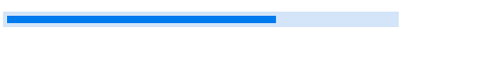## Color Customization

You can customize the color of the linear progressbar’s progress color and track color. The following properties are used to customize the color in the progressbar.

ProgressColor: Represents the color of the progress indicator.

TrackColor: Represents the color of the track indicator.

``<Syncfusion:SfLinearProgressBar Progress="70" ProgressColor="LawnGreen" TrackColor="DarkOliveGreen"/>``
``````SfLinearProgressBar linear = new SfLinearProgressBar();
linear.Progress = 70;
linear.ProgressColor = new SolidColorBrush(Colors.LawnGreen);
linear.TrackColor = new SolidColorBrush(Colors.DarkOliveGreen);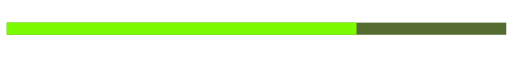## Range Colors

You can visualize multiple ranges with different solid colors that are mapped to each range to enhance the readability of progress.
The solid colors can be mapped to the specific ranges using the RangeColor.

Color: Represents the color to the specified range.

Start: Represents the start range of the color.

End: Represents the end range of the color.
You can visualize the multiple ranges with different solid colors that are mapped to each range to enhance the readability of progress.

``````<Syncfusion:SfLinearProgressBar Progress="80">
<Syncfusion:SfLinearProgressBar.RangeColors>
<Syncfusion:RangeColorCollection>
<Syncfusion:RangeColor Color="BlanchedAlmond" Start="10" End="30"/>
<Syncfusion:RangeColor Color="Coral" Start="30"  End="60"/>
<Syncfusion:RangeColor Color="Crimson" Start="60" End="100"/>
</Syncfusion:RangeColorCollection>
</Syncfusion:SfLinearProgressBar.RangeColors>
</Syncfusion:SfLinearProgressBar>``````
``````SfLinearProgressBar linear = new SfLinearProgressBar();
RangeColorCollection rangeColors = new RangeColorCollection();
rangeColors.Add(new RangeColor() { Color = Colors.BlanchedAlmond, Start = 5, End = 30 });
rangeColors.Add(new RangeColor() { Color = Colors.Coral, Start = 30, End = 60 });
rangeColors.Add(new RangeColor() { Color = Colors.Crimson, Start = 60, End = 100 });
linear.RangeColors = rangeColors;
linear.Progress = 80;
linear.Width = 500;
linear.Height = 20;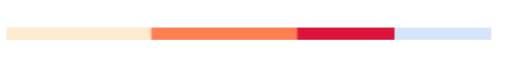Gradient shows change in intensity of the colors during the progress. IsGradient property in RangeColor class to get the gradient effect in the colors applied to the progressbar.

``````<Syncfusion:SfLinearProgressBar Progress="80>
<Syncfusion:SfLinearProgressBar.RangeColors>
<Syncfusion:RangeColorCollection>
</Syncfusion:RangeColorCollection>
</Syncfusion:SfLinearProgressBar.RangeColors>
</Syncfusion:SfLinearProgressBar>``````
``````SfLinearProgressBar linear = new SfLinearProgressBar();
RangeColorCollection rangeColors = new RangeColorCollection();
rangeColors.Add(new RangeColor() { IsGradient=true, Color =   Colors.SkyBlue, Start = 10, End = 30 });
rangeColors.Add(new RangeColor() { IsGradient = true,Color =  Colors.DeepSkyBlue, Start = 30, End = 60 });
rangeColors.Add(new RangeColor() { IsGradient = true,Color =  Colors.Blue, Start = 60, End = 100 });
linear.RangeColors = rangeColors;
linear.Progress = 80;
linear.Width = 500;
linear.Height = 20;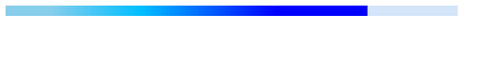## AnimationDuration

You can customize the duration for completing one animation cycle and it applies when the `IsIndeterminate` is true. The default value is `3000ms.`

``````<Grid Name="grid">
<Syncfusion:SfLinearProgressBar
Width="250"
Height="4"
AnimationDuration="00:00:01"
IsIndeterminate="True"
Progress="50" />
</Grid>``````
``````SfLinearProgressBar linear = new SfLinearProgressBar();
linear.Progress = 50;
linear.IsIndeterminate = true;
linear.Width = 250;
linear.Height = 4;
linear.AnimationDuration= new TimeSpan(0,0,1);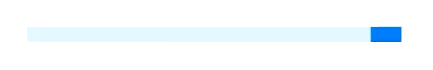## AnimationEasing

You can customize the easing function to apply for the linear and circular progress bar animation and it applies when the `IsIndeterminate` is true.

``````<Grid Name="grid">
<Syncfusion:SfLinearProgressBar
Width="250"
Height="4"
IsIndeterminate="True"
Progress="50">
<Syncfusion:SfLinearProgressBar.AnimationEasing>
<BounceEase
Bounces="20"
Bounciness="5"
EasingMode="EaseOut" />
</Syncfusion:SfLinearProgressBar.AnimationEasing>
</Syncfusion:SfLinearProgressBar>
</Grid>``````
``````SfLinearProgressBar linear = new SfLinearProgressBar();
linear.Progress = 50;
linear.IsIndeterminate = true;
linear.Width = 250;
linear.Height = 4;
BounceEase bounceEase = new BounceEase();
bounceEase.Bounces = 20;
bounceEase.Bounciness = 5;
bounceEase.EasingMode = EasingMode.EaseOut;
linear.AnimationEasing = bounceEase;